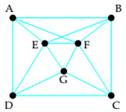# Use Dirac's theorem to verify that the graph is Hamiltonian. Then find a Hamiltonian circuit.### Mathematical Excursions (MindTap C...

4th Edition
Richard N. Aufmann + 3 others
Publisher: Cengage Learning
ISBN: 9781305965584

#### Solutions

Chapter
Section### Mathematical Excursions (MindTap C...

4th Edition
Richard N. Aufmann + 3 others
Publisher: Cengage Learning
ISBN: 9781305965584
Chapter 5.2, Problem 1ES
Textbook Problem
262 views

## Use Dirac's theorem to verify that the graph is Hamiltonian. Then find a Hamiltonian circuit.To determine

To determine if the graph is Hamiltonian using Dirac's theorem. Then to find a Hamiltonian circuit.

### Explanation of Solution

Given information:

Given, graph is:

Calculation:

Dirac's theorem states, for a connected graph with at least three vertices and no multiple edges, if the number of vertices is n and every vertex has degree of at least n/2, then the graph is a Hamiltonian graph.

Here,

The number of vertices in the graph is 7 (A, B, C, D, E, F, G)

So the graph is Hamiltonian if the degree of every vertex is at least 7/2=3...

### Still sussing out bartleby?

Check out a sample textbook solution.

See a sample solution

#### The Solution to Your Study Problems

Bartleby provides explanations to thousands of textbook problems written by our experts, many with advanced degrees!

Get Started

Find more solutions based on key concepts
In problems 19-22, rewrite the expression with positive exponents. 20.

Mathematical Applications for the Management, Life, and Social Sciences

The circumference of a circle is given by C(r) = 2r where r is the radius of the circle. What is the circumfere...

Applied Calculus for the Managerial, Life, and Social Sciences: A Brief Approach

In Exercises 11-14, factor the expression. x23x+2

Calculus: An Applied Approach (MindTap Course List)

Solve the equations in Exercises 126. (x+1)(x+2)2+(x+1)2(x+2)=0

Finite Mathematics and Applied Calculus (MindTap Course List)

Sketching a Surface in Space In Exercises 49-58, describe and sketch the surface. y=z2

Calculus: Early Transcendental Functions (MindTap Course List)

True or False: xy + 6x2y = 10 x3 is linear.

Study Guide for Stewart's Single Variable Calculus: Early Transcendentals, 8th

In problem 1518 use the method of Example 2 to compute eAt for the coefficient matrix. Use (1) to find the gene...

A First Course in Differential Equations with Modeling Applications (MindTap Course List)

In recent years more people have been working past the age of 65. In 2005, 27% of people aged 65-69 worked. A r...

Modern Business Statistics with Microsoft Office Excel (with XLSTAT Education Edition Printed Access Card) (MindTap Course List)

The Skydiver Again When a skydiver jumps from an airplane, her downward velocity, n feet per second, before she...

Functions and Change: A Modeling Approach to College Algebra (MindTap Course List)